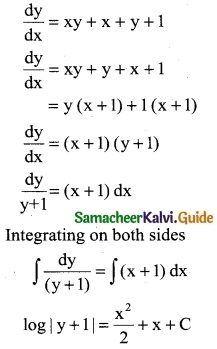Tamilnadu State Board New Syllabus Samacheer Kalvi 12th Business Maths Guide Pdf Chapter 4 Differential Equations Miscellaneous Problems Text Book Back Questions and Answers, Notes.

## Tamilnadu Samacheer Kalvi 12th Business Maths Solutions Chapter 4 Differential Equations Miscellaneous Problems

Question 1.
Suppose that Qd = 30 – 5P + 2$$\frac { dp }{dt}$$ + $$\frac { d^2p }{dt^2}$$ and Qs = 6 + 3P Find the equilibrium price for market clearance.
Solution :
Qd = 30 – 5P + 2$$\frac { dp }{dt}$$ + $$\frac { d^2p }{dt^2}$$ and
Qs = 6 + 3P
For market clearance, the required condition is
Qd = Qs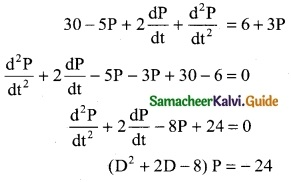The auxiliary equation is m2 + 2m – 8 = 0
(m + 4) (m – 2) = 0
m = -4, 2
Roots are real and different
C.F = Aem1x + Bem2x
C.F = Ae-4t + Be2t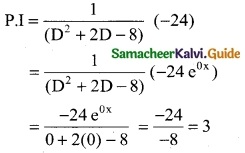The general solution is y = C.F + P.I
y = Ae-ut + Be2t + 3Question 2.
Form the differential equation having for its general solution y = ax² + bx
Solution:
y = ax² + bx ……….. (1)
Since we have two arbitary constants, differentiative twice.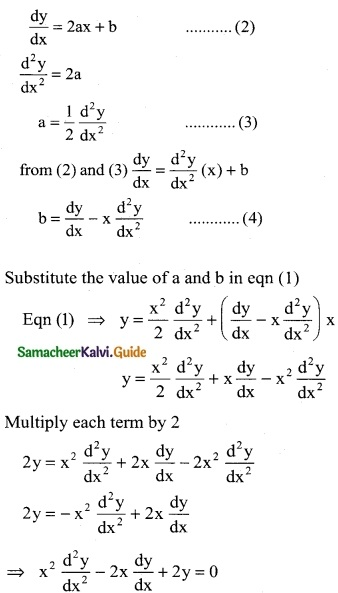Question 3.
Solve yx²dx + e-x dy = 0
Solution:
yx²dx + e-x dy = 0
e-x dy = -yx²dx
$$\frac { 1 }{y}$$ dy = -x² ex dx
Integrating on both sides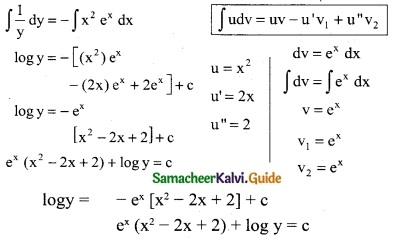Question 4.
Solve (x² + y²) dx + 2xy dy = 0
Solution:
(x² + y²) dx + 2xy dy = 0
2xy dy = – (x² + y²) dx
$$\frac { dy }{dx}$$ = $$\frac { -(x^2+y^2) }{2xy}$$ ………. (1)
This is a homogeneous differential equation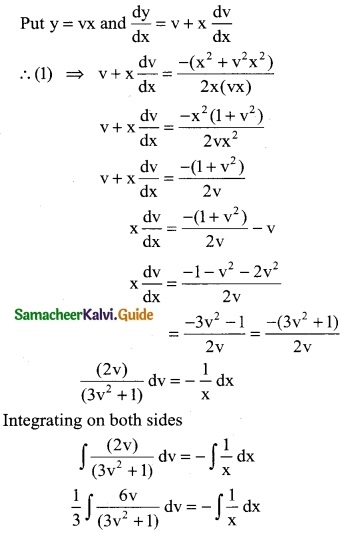$$\frac { 1 }{3}$$ log(3v² + 1) = – logx + logc
log (3v² + 1)1/3 + log x = log c
logx (3v² + 1)1/3 = log c
⇒ x (3v² + 1)1/3 = c
⇒ x[$$\frac { 3y^2 }{x^2}$$ + 1]1/3 = c

Question 5.
Solve x $$\frac { dy }{dx}$$ + 2y = x4
Solution: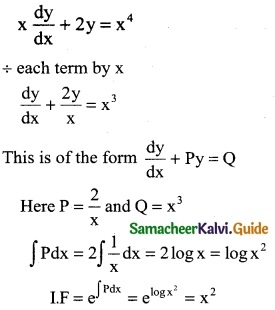This solution is
y(I.F) = ∫Qx (I.F) dx + c
y(x²) = ∫(x³ × x²) dx + c
yx² = ∫x5 dx + c
⇒ yx² = $$\frac { x^6 }{6}$$ + cQuestion 6.
A manufacturing company has found that the cost C of operating and maintaining the equipment is related to the length’m’ of intervals between overhauls by the equation m² $$\frac { dc }{dm}$$ + 2mc = 2 and c = 4 and whem m = 2. Find the relationship between C and m.
Solution:
m² $$\frac { dc }{dm}$$ + 2mc = 2
÷ each term by m²
$$\frac { dc }{dm}$$ + $$\frac { 2mc }{m^2}$$ = $$\frac { 2 }{m^2}$$
$$\frac { dc }{dm}$$ + $$\frac { 2c }{m}$$ = $$\frac { 2 }{m^2}$$
This is a first order linear differential equation of the form
$$\frac { dc }{dm}$$ + Pc = Q where P = $$\frac { 2 }{m}$$ and Q = $$\frac { 2 }{m^2}$$
∫Pdm = 2 ∫$$\frac { 1 }{m}$$dm = 2 log m = log m²
I.F = e∫Pdm = elogm² = m²
General solution is
C (I.F) = ∫Q × (IF) dm + k
C(m²) = ∫$$\frac { 2 }{m^2}$$ × (m²) dm + k
C(m²) = ∫2dm + k
Cm² = 2m + k ……….. (1)
when C = 4 and m = 2, we have
(4) (2)² = 2(2) + k
16 = 4 + k = 12
Equation (1)
Cm² = 2m + 12
Cm² = 2(m + 6)Question 7.
Solve (D² – 3D + 2) y = e4x
Solution:
(D² – 3D + 2) y = e4x
The auxiliary equation is m² – 3m + 2 = 0
(m – 1) (m – 2) = 0
m = 1, 2
The roots are real and different
C.F = Aem1x + Bem1x
C.F = Aex + Be2x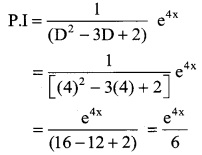The general solution is y = C.F + P.I
y = Aex + Be2x + $$\frac { e^{4x} }{6}$$ ………. (1)
When x = 0; y = 0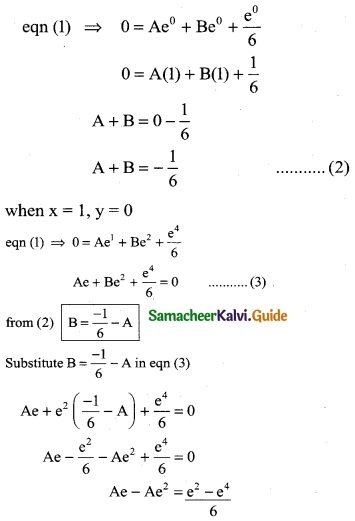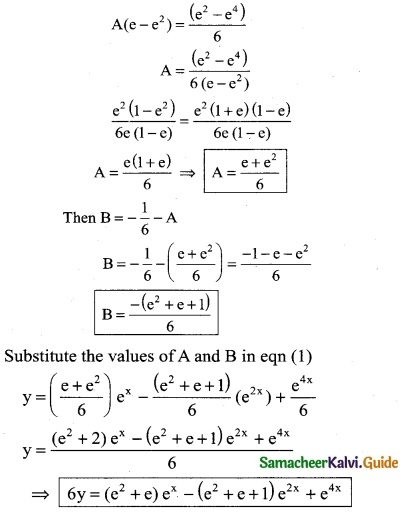Question 8.
Solve $$\frac { dy }{dx}$$ + y cos x = 2 cos x
Solution:
$$\frac { dy }{dx}$$ + y cos x = 2 cos x
This is of the form $$\frac { dy }{dx}$$ + Py = Q
Here P = cos x and Q = 2 cos x
∫Pdx = ∫cos x dx = sin x
I.F = e∫pdx = esin x
The solution is
y (I.F) = ∫Q (I.F) dx + c
yesin x = ∫(2 cos x) esin x dx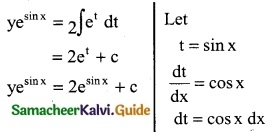Question 9.
Solve x²ydx – (x³ + y³) dy = 0
Solution:
x²ydx = (x³ + y³) dy = 0
x²ydx = (x³ + y³) dy
$$\frac { dy }{dx}$$ = $$\frac { x^2y }{(x^3+y^3)}$$ ……… (1)
This is a homogeneous differential equation, same degree in x and y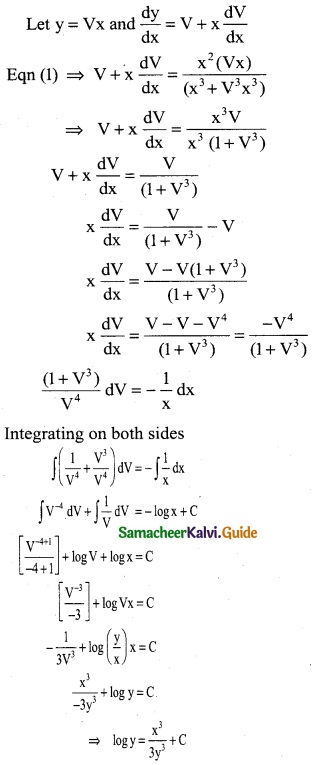Question 10.
Solve $$\frac { dy }{dx}$$ = xy + x + y + 1
Solution: